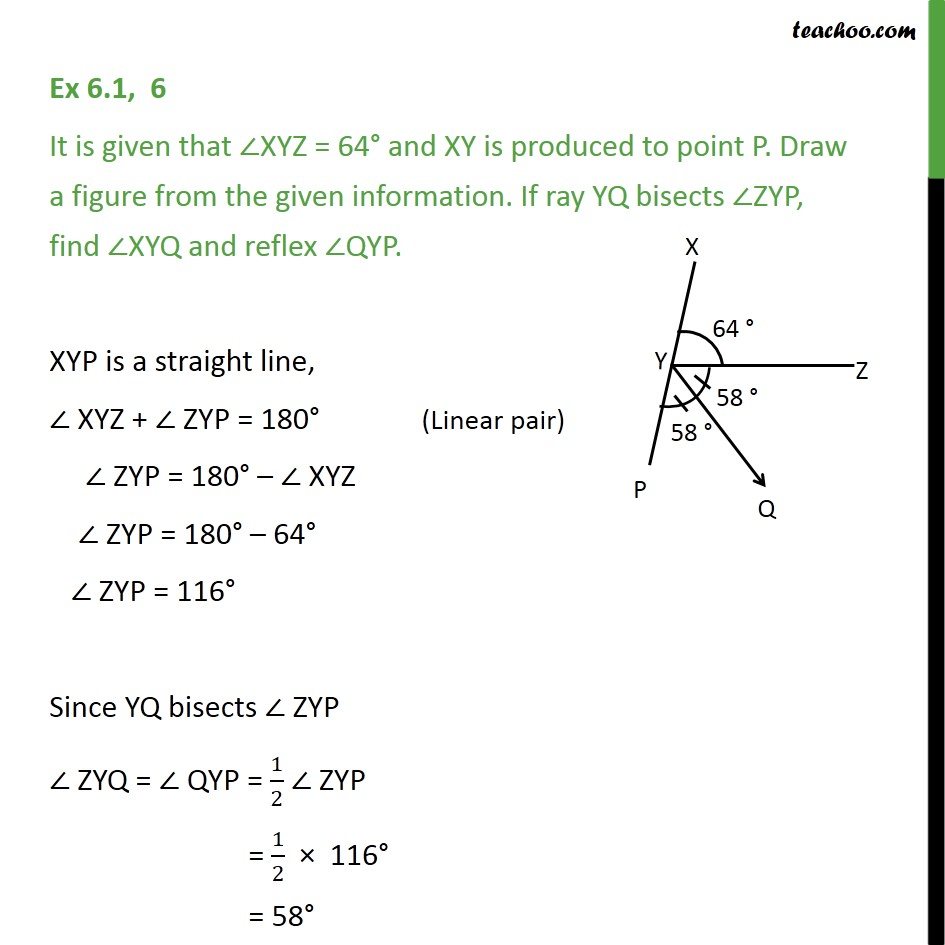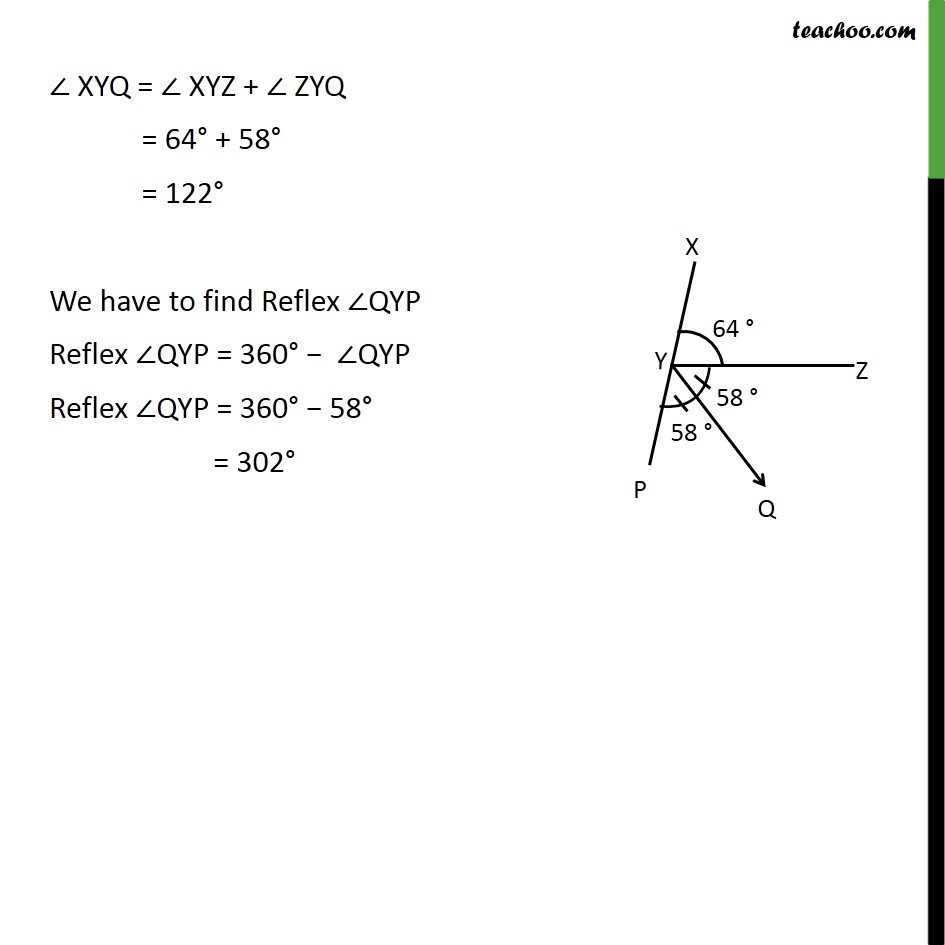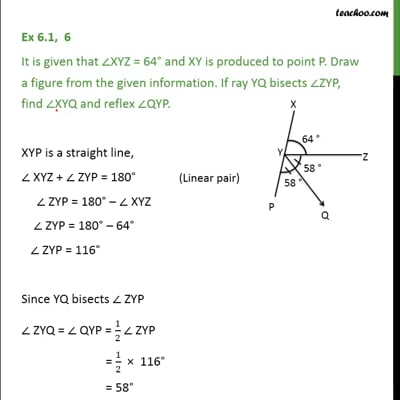Ex 6.1

Chapter 6 Class 9 Lines and Angles
Serial order wiseThis video is only available for Teachoo black users

Maths Crash Course - Live lectures + all videos + Real time Doubt solving!

### Transcript

Ex 6.1, 6 It is given that XYZ = 64 and XY is produced to point P. Draw a figure from the given information. If ray YQ bisects ZYP, find XYQ and reflex QYP. XYP is a straight line, XYZ + ZYP = 180 ZYP = 180 XYZ ZYP = 180 64 ZYP = 116 Since YQ bisects ZYP ZYQ = QYP = 1/2 ZYP = 1/2 116 = 58 XYQ = XYZ + ZYQ = 64 + 58 = 122 We have to find Reflex QYP Reflex QYP = 360 QYP Reflex QYP = 360 58 = 302